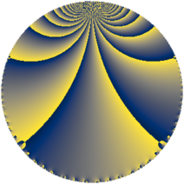# Properties

 Label 225.4.pLevel $225$ Weight $4$ Character orbit 225.p Rep. character $\chi_{225}(32,\cdot)$ Character field $\Q(\zeta_{12})$ Dimension $208$ Newform subspaces $3$ Sturm bound $120$ Trace bound $1$

# Related objects

## Defining parameters

 Level: $$N$$ $$=$$ $$225 = 3^{2} \cdot 5^{2}$$ Weight: $$k$$ $$=$$ $$4$$ Character orbit: $$[\chi]$$ $$=$$ 225.p (of order $$12$$ and degree $$4$$) Character conductor: $$\operatorname{cond}(\chi)$$ $$=$$ $$45$$ Character field: $$\Q(\zeta_{12})$$ Newform subspaces: $$3$$ Sturm bound: $$120$$ Trace bound: $$1$$ Distinguishing $$T_p$$: $$2$$

## Dimensions

The following table gives the dimensions of various subspaces of $$M_{4}(225, [\chi])$$.

Total New Old
Modular forms 384 224 160
Cusp forms 336 208 128
Eisenstein series 48 16 32

## Trace form

 $$208 q + 6 q^{2} + 24 q^{6} + 2 q^{7} + O(q^{10})$$ $$208 q + 6 q^{2} + 24 q^{6} + 2 q^{7} + 36 q^{11} + 138 q^{12} + 2 q^{13} + 1468 q^{16} + 480 q^{18} - 984 q^{21} + 34 q^{22} - 306 q^{23} - 180 q^{27} + 232 q^{28} - 4 q^{31} + 1770 q^{32} + 294 q^{33} - 360 q^{36} - 136 q^{37} - 114 q^{38} - 4020 q^{41} - 1698 q^{42} + 2 q^{43} + 1856 q^{46} - 3462 q^{47} - 4326 q^{48} - 672 q^{51} + 242 q^{52} + 14220 q^{56} + 2544 q^{57} - 534 q^{58} - 76 q^{61} + 4038 q^{63} - 8520 q^{66} - 610 q^{67} + 2694 q^{68} + 1854 q^{72} + 8 q^{73} - 1980 q^{76} + 6486 q^{77} - 1434 q^{78} - 7968 q^{81} + 3784 q^{82} - 2814 q^{83} - 14556 q^{86} - 4830 q^{87} + 1338 q^{88} - 2032 q^{91} - 13152 q^{92} - 8310 q^{93} + 17664 q^{96} - 358 q^{97} + O(q^{100})$$

## Decomposition of $$S_{4}^{\mathrm{new}}(225, [\chi])$$ into newform subspaces

Label Dim $A$ Field CM Traces $q$-expansion
$a_{2}$ $a_{3}$ $a_{5}$ $a_{7}$
225.4.p.a $48$ $13.275$ None $$0$$ $$0$$ $$0$$ $$0$$
225.4.p.b $64$ $13.275$ None $$6$$ $$0$$ $$0$$ $$2$$
225.4.p.c $96$ $13.275$ None $$0$$ $$0$$ $$0$$ $$0$$

## Decomposition of $$S_{4}^{\mathrm{old}}(225, [\chi])$$ into lower level spaces

$$S_{4}^{\mathrm{old}}(225, [\chi]) \cong$$ $$S_{4}^{\mathrm{new}}(45, [\chi])$$$$^{\oplus 2}$$